• AGNES(Agglomerative Nesting) 是凝聚的层次聚类算法，如果簇C1中一个对象和簇C2中一个对象之间距离是所有属于不同簇对象间欧式距离中最小，C1和C2可能被合并。这是一种单连接方法，其每个簇可以被簇中...
文章目录层次聚类距离度量凝聚层次聚类算法描述：优劣：codepythonmatlab
层次聚类
距离度量
层次聚类算法有多种，它们的步骤基本相同，差别在于聚类间距的定义不
同。计算聚类距离间距的计算方法主要有: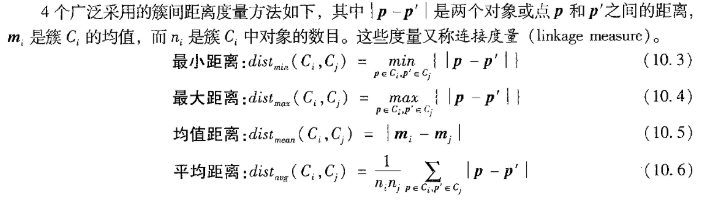凝聚层次聚类
AGNES(Agglomerative Nesting) 是凝聚的层次聚类算法，如果簇C1中的一个对象和簇C2中的一个对象之间的距离是所有属于不同簇的对象间欧式距离中最小的，C1和C2可能被合并。这是一种单连接方法，其每个簇可以被簇中的所有对象代表，两个簇之间的相似度由这两个簇中距离最近的数据点对的相似度来确定。
合并型层次聚类及产生二分树图的基本步骤如下：

计算n个对象两两之间的距离。
构造n个单成员聚类$C_1, C_2,..., C_n$。，每一类的高度都为0。
找到两个最近的聚类$C_i，C_j$; 合并$C_i，C_j$;，聚类的个数减少1,以被合并的两个类间距作为上层的高度。
计算新生成的聚类与本层中其他聚类的间距，如果满足终止条件，算法结束，否则转(3)。

算法描述：
input：包含n个对象的数据库，终止条件簇的数目k
output：k个簇
（1）       将每个对象当成一个初始簇
（2）       Repeat
（3）                根据两个簇中最近的数据点找到最近的两个簇
（4）                合并两个簇，生成新的簇的集合
（5）       Until达到定义的簇的数目

优劣：

简单，但遇到合并点选择困难的情况。
一旦一组对象被合并，不能撤销
算法的复杂度为O(n的平方)，不适合大数据集

code
python
def euler_distance(point1: np.ndarray, point2: list) -> float:
"""
计算两点之间的欧拉距离，支持多维
"""
distance = 0.0
for a, b in zip(point1, point2):
distance += math.pow(a - b, 2)
return math.sqrt(distance)

class ClusterNode(object):
def __init__(self, vec, left=None, right=None, distance=-1, id=None, count=1):
"""
:param vec: 保存两个数据聚类后形成新的中心
:param left: 左节点
:param right:  右节点
:param distance: 两个节点的距离
:param id: 用来标记哪些节点是计算过的
:param count: 这个节点的叶子节点个数
"""
self.vec = vec
self.left = left
self.right = right
self.distance = distance
self.id = id
self.count = count

class Hierarchical(object):
def __init__(self, pp, k = 1 ):
# Python assert（断言）用于判断一个表达式，在表达式条件为 false 的时候触发异常。
# 断言可以在条件不满足程序运行的情况下直接返回错误
assert k > 0
# k 聚类数目
self.k = k
# 标签
self.labels = None
# 预处理的数据处理类
self.pp = pp

# 数据表 行为记录
# [
#   [1,2,...],
#   [1,2,...],
#   ...
# ]
def fit(self, x):
# enumerate() 函数用于将一个可遍历的数据对象(如列表、元组或字符串)
# 组合为一个索引序列，同时列出数据 v 和数据下标 i
# 初始化所有节点（将所有的实例都当作一个节点）
nodes = [ClusterNode(vec=v, id=i) for i, v in enumerate(x)]
# 距离字典
# {
#   (node1,node2):distance,
#   (node1,node3):distance,
#   ...
# }
distances = {}
# 特征的维度
#   point_num：实例数目（贝叶斯网络中就是变量数目）
#   future_num ：特征（属性）数目（贝叶斯网络中就是实例数目）
point_num, future_num = np.shape(x)
# 初始化特征
self.labels = [ -1 ] * point_num
# 初试化当前节点ID
currentclustid = -1
# 进入凝聚的步骤 第一次循环：每条实例当作一个簇
# 截止条件：nodes中节点数目不大于 K
while len(nodes) > self.k:
# 初始化最小距离为极大值
min_dist = math.inf
# 节点数目
nodes_len = len(nodes)
# 表示最相似的两个聚类
closest_part = None
# 双层循环计算 距离
for i in range(nodes_len - 1):
for j in range(i + 1, nodes_len):
# 为了不重复计算距离，保存在字典内
d_key = (nodes[i].id, nodes[j].id)
if d_key not in distances:
distances[d_key] = euler_distance(nodes[i].vec, nodes[j].vec)
d = distances[d_key]
# 获取所有节点之间的最短距离 和节点对信息
if d < min_dist:
min_dist = d
closest_part = (i, j)
# 合并两个聚类 part1, part2是节点的ID
part1, part2 = closest_part
node1, node2 = nodes[part1], nodes[part2]
# 构建新的节点中心=======================
new_vec = [(node1.vec[ind] * node1.count + node2.vec[ind] * node2.count)
/ (node1.count + node2.count)
for ind in range(future_num)]
# 构建新的节点
new_node = ClusterNode(vec=new_vec,
left=node1,
right=node2,
distance=min_dist,
id=currentclustid,
count=node1.count + node2.count)
currentclustid -= 1
# 一定要先del索引较大的
del nodes[part2], nodes[part1]
# 新节点加入到nodes容器中
nodes.append(new_node)
self.nodes = nodes
# 打标签
self.calc_label()

def calc_label(self):
"""
调取聚类的结果
"""
for i, node in enumerate(self.nodes):
# 将节点的所有叶子节点都分类
self.leaf_traversal(node, i)

def leaf_traversal(self, node: ClusterNode, label):
"""
递归遍历叶子节点
"""
if node.left == None and node.right == None:
self.labels[node.id] = label
if node.left:
self.leaf_traversal(node.left, label)
if node.right:
self.leaf_traversal(node.right, label)

pp = PreProcess()
pp.uses()

# print(type(iris.data),iris)
my = Hierarchical(pp, 25)
# my.fit(iris.data)
my.fit(pp.ndarray())
pp.printVar()
print(np.array(my.labels))
my.calc_label()

matlab
K_cluster = 8;
%% 初始化底层节点
node = generate_node();
% 构建初始节点
nodes = init_nodes(node, varSize);
% 当做非叶子节点的id（没什么用）
currentclustid = -1;
%% j
while length(nodes) > K_cluster
len_nodes = length(nodes);

min_dist = -inf;
min_pair_node = [];
%% 选出最近的两个节点
for ind =1:len_nodes
for jnd =(ind+1):len_nodes
node_A = nodes(ind);
node_B = nodes(jnd);
distance = average_distance(node_A, node_B, MMatrix);
% 这儿是互信息，越大越好
if distance > min_dist
min_dist = distance;
% 记录
min_pair_node = [ind jnd];
end
end
end
%% 合并最近的两个节点
ind = min_pair_node(1);
jnd = min_pair_node(2);
node_A = nodes(ind);
node_B = nodes(jnd);
% 构建新的节点
new_node = merge_two_node(node_A, node_B,distance,currentclustid);
% 删除nodes中的node_A,node_B
nodes(ind) = [];
% ind位置删除之后，jnd需要减一位
nodes(jnd-1) = [];
% 添加 new_node 到 nodes
nodes(length(nodes)+1) = new_node;
% nodes = [nodes [new_node]];
% 更新节点
currentclustid = currentclustid -1;
end

function nodes = init_nodes(node, varSize)
% 模板节点 node
% 变量数目
% 根据一个节点模板生成 varSize 个叶子节点
nodes = repmat(node, varSize, 1);
for ind =1:varSize
nodes(ind).vec = [ind];
nodes(ind).left = [];
nodes(ind).right = [];
nodes(ind).distance = 0;
nodes(ind).id = ind;
nodes(ind).count = 1;
nodes(ind).leafnode = [ind];
end
end

function node = generate_node()
% 保存两个数据聚类后形成新的中心
node.vec = [];
% 左节点
node.left = [];
% 右节点
node.right = [];
% 左右两个节点的距离
node.distance = [];
% 用来标记哪些节点是计算过的
node.id = [];
% 这个节点的叶子节点个数（叶子节点的数目）
node.count = [];
% 这个节点的所以叶子节点（叶子节点集合）
node.leafnode = [];
end

function node = merge_two_node(node_A, node_B,distance,currentclustid)
% 保存两个数据聚类后形成新的中心
% node = generate_node();
node.vec = [];
% 左节点
node.left = node_A;
% 右节点
node.right = node_B;
% 左右两个节点的距离
node.distance = distance;
% 用来标记哪些节点是计算过的
node.id = currentclustid;
% 这个节点的叶子节点个数（叶子节点的数目）
node.count = node_A.count + node_B.count ;
% 这个节点的所以叶子节点（叶子节点集合）
node.leafnode = [node_A.leafnode  node_B.leafnode];
end

function distance = average_distance(node_A, node_B, MMatrix)
% Average distance
%   A 节点
%   B 节点
%   距离矩阵
sum_distance = 0;
% 计算 sum distance
lenA = length(node_A.leafnode) ;
lenB = length(node_B.leafnode);
% 计算平均距离
for i=1:lenA
for j=1:lenB
X = node_A.leafnode(i);
Y = node_B.leafnode(j);
dist = MMatrix(X,Y);
sum_distance = sum_distance + dist;
end
end
%   数  目
n_i_n_j = node_A.count*node_B.count;
distance = sum_distance/n_i_n_j;
end



展开全文聚类 凝聚聚类 cluster
• 这篇博客开始另外一种聚类——层次聚类，层次聚类和K-Means是同一类属于划分聚类。层次聚类方法对给定数据集进行层次分解，直到满足某种条件为止.
这篇博客开始另外一种聚类——层次聚类，层次聚类和K-Means是同一类的，属于划分聚类。
概述
层次聚类方法对给定的数据集进行层次的分解，直到满足某种条件为止，传统的层次聚类算法主要分为两大类算法：

凝聚的层次聚类：AGNES算法( AGglomerative NESting )=>采用自底向上的策略。 最初将每个对象作为一个簇，然后这些簇根据某些准则被一步一步合并，两个簇间的距离可以由这两个不同簇中距离最近的数据点的相似度来确定；聚类的合并过程反复进行直到所有的对象满足簇数目。
分裂的层次聚类：DIANA算法(DIvisive ANALysis)=>采用自顶向下的策略（与二分k-means非常类似）。首先将所有对象置于一个簇中，然后按照某种既定的规则（聚类规则，如k-means）逐渐细分为越来越小的簇(比如最大的欧式距离)，直到达到某个终结条件(簇数目或者簇距离达到阈值)。

AGNES和DIANA算法优缺点

简单，理解容易
合并点/分裂点选择不太容易
合并/分类的操作不能进行撤销
大数据集不太适合
执行效率较低 $O(t*n^2)$，t为迭代次数，n为样本点数

接下来着重看一下AGNES算法中簇间距离的合并策略

最小距离(SL聚类)
最终得到模型容易形成链式结构
最大距离(CL聚类)
如果存在异常值，那么构建可能不太稳定
平均距离(AL聚类)
不同的合并策略

代码
当然这里也只是以AGNES为例。
API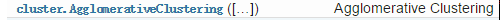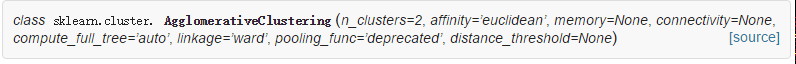参数

n_clusters：聚类数
affinity : string or callable, default: “euclidean”，距离度量公式，默认为欧式
memory：内存或磁盘文件，因为计算量比较大，比较耗内存
linkage : {“ward”, “complete”, “average”, “single”}, optional (default=”ward”)，相似度度量方式

代码
import numpy as np
import matplotlib as mpl
import matplotlib.pyplot as plt
from sklearn.cluster import AgglomerativeClustering
from sklearn.neighbors import kneighbors_graph ## KNN的K近邻计算
import sklearn.datasets as ds
import warnings

## 设置属性防止中文乱码及拦截异常信息
mpl.rcParams['font.sans-serif'] = [u'SimHei']
mpl.rcParams['axes.unicode_minus'] = False
warnings.filterwarnings(action='ignore', category=UserWarning)

## 模拟数据产生: 产生600条数据
np.random.seed(0)
n_clusters = 4
N = 1000
data1, y1 = ds.make_blobs(n_samples=N, n_features=2, centers=((-1, 1), (1, 1), (1, -1), (-1, -1)), random_state=0)

n_noise = int(0.1*N)
r = np.random.rand(n_noise, 2)
min1, min2 = np.min(data1, axis=0)
max1, max2 = np.max(data1, axis=0)
r[:, 0] = r[:, 0] * (max1-min1) + min1
r[:, 1] = r[:, 1] * (max2-min2) + min2

data1_noise = np.concatenate((data1, r), axis=0)
y1_noise = np.concatenate((y1, *n_noise))
#拟合月牙形数据
data2, y2 = ds.make_moons(n_samples=N, noise=.05)
data2 = np.array(data2)
n_noise = int(0.1 * N)
r = np.random.rand(n_noise, 2)
min1, min2 = np.min(data2, axis=0)
max1, max2 = np.max(data2, axis=0)
r[:, 0] = r[:, 0] * (max1 - min1) + min1
r[:, 1] = r[:, 1] * (max2 - min2) + min2
data2_noise = np.concatenate((data2, r), axis=0)
y2_noise = np.concatenate((y2,  * n_noise))

def expandBorder(a, b):
d = (b - a) * 0.1
return a-d, b+d

## 画图
# 给定画图的颜色
cm = mpl.colors.ListedColormap(['#FF0000', '#00FF00',  '#0000FF', '#d8e507', '#F0F0F0'])
plt.figure(figsize=(14, 12), facecolor='w')
for index, (n_clusters, data, y) in enumerate(((4, data1, y1), (4, data1_noise, y1_noise),
(2, data2, y2), (2, data2_noise, y2_noise))):
# 前面的两个4表示几行几列，第三个参数表示第几个子图(从1开始，从左往右数)
plt.subplot(4, 4, 4*index+1)
plt.scatter(data[:, 0], data[:, 1], c=y, cmap=cm)
plt.title(u'原始数据', fontsize=17)
plt.grid(b=True, ls=':')
min1, min2 = np.min(data, axis=0)
max1, max2 = np.max(data, axis=0)
plt.xlim(expandBorder(min1, max1))
plt.ylim(expandBorder(min2, max2))

# 计算类别与类别的距离(只计算最接近的七个样本的距离) -- 希望在agens算法中，在计算过程中不需要重复性的计算点与点之间的距离
connectivity = kneighbors_graph(data, n_neighbors=7, mode='distance', metric='minkowski', p=2, include_self=True)
connectivity = (connectivity + connectivity.T)
##进行建模，并传值
print(n_clusters)
ac = AgglomerativeClustering(n_clusters=n_clusters, affinity='euclidean',
ac.fit(data)
y = ac.labels_

plt.subplot(4, 4, i+2+4*index)
plt.scatter(data[:, 0], data[:, 1], c=y, cmap=cm)
plt.grid(b=True, ls=':')
plt.xlim(expandBorder(min1, max1))
plt.ylim(expandBorder(min2, max2))

plt.suptitle(u'AGNES层次聚类的不同合并策略', fontsize=30)
plt.tight_layout(0.5, rect=(0, 0, 1, 0.95))
plt.show()


4
4
4
4
4
4
2
2
2
2
2
2

最后看一下效果图：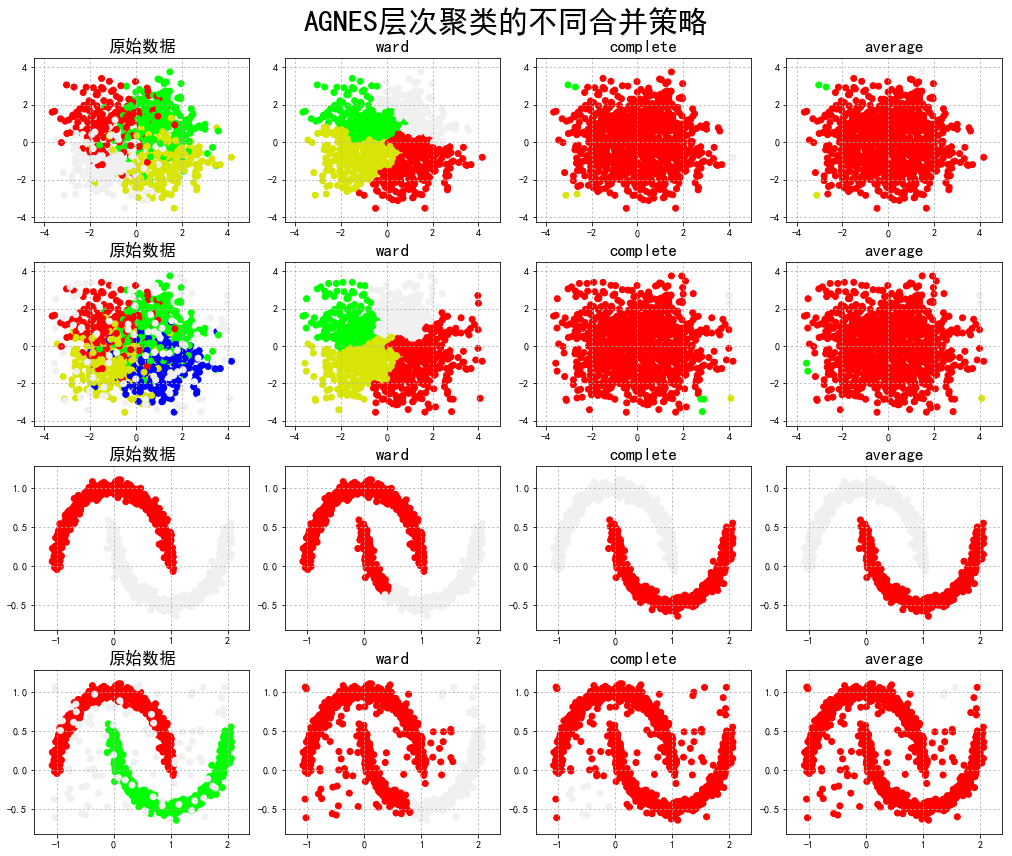展开全文机器学习 聚类 DIANA
• 系统聚类原理 ...Divisive 层次聚类：又称自顶向下（top-down）的层次聚类，最开始所有对象均属于一个cluster，每次按一定准则将某个cluster 划分为多个cluster，如此往复，直至每个对象均是一个
系统聚类原理
层次聚类(Hierarchical Clustering)是聚类算法的一种，通过计算不同类别数据点间的相似度来创建一棵有层次的嵌套聚类树。在聚类树中，不同类别的原始数据点是树的最低层，树的顶层是一个聚类的根节点。创建聚类树有自下而上合并和自上而下分裂两种方法。
层次聚类算法一般分为两类：
Divisive 层次聚类：又称自顶向下（top-down）的层次聚类，最开始所有的对象均属于一个cluster，每次按一定的准则将某个cluster 划分为多个cluster，如此往复，直至每个对象均是一个cluster。
Agglomerative 层次聚类：又称自底向上（bottom-up）的层次聚类，每一个对象最开始都是一个cluster，每次按一定的准则将最相近的两个cluster合并生成一个新的cluster，如此往复，直至最终所有的对象都属于一个cluster。
图片来自https://www.biaodianfu.com/hierarchical-clustering.html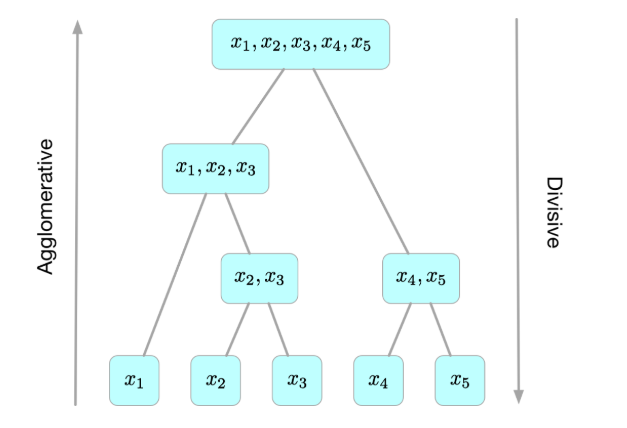这个算法原理很简单，实现也不难

scala
class Hierarchical(var T: Double //聚类个数
, var data:List[Array[Double]] //数据集

) {
var finalResult = initFinalResult
// data=standardization01(data)
var initialList=data//初始列表

var firstCluster=new ListBuffer[Int]()//首次出现聚类的列表
var nextCluster=new ListBuffer[Array[Int]]()//首次出现的位置(聚类1和聚类2)
var table=new ListBuffer[Array[Double]]()//表格
var clusterHistory=ListBuffer[ListBuffer[ListBuffer[Array[Double]]]]()//迭代历史
var stage=0;
//初始化，N个初始模式样本自成一类
private def initFinalResult:ListBuffer[ListBuffer[Array[Double]]] = {
val startResult =new ListBuffer[ListBuffer[Array[Double]]]
//首先将每一样本看成单独一类
val list=new ListBuffer[Array[Double]]
startResult.append(list)
}
startResult
}

def hierarchical: ListBuffer[ListBuffer[Array[Double]]] = {
if (finalResult.size == 1) return finalResult
//计算每类间的欧式距离，保存在二维数组中
var distanceArray =Array.ofDim[Double](finalResult.size,finalResult.size)
//最短距离 初始化为1,2类的距离
// var min_dis = min_distance(finalResult(0), finalResult(1))
//组间连接法
var min_dis=baverage_distance(finalResult(0),finalResult(1))
//即将合并的类的标号
var index1 = 0
var index2 = 1
for (i <- 0 until finalResult.size) {
for (j <- (i + 1) until finalResult.size) {
distanceArray(i)(j) = baverage_distance(finalResult(i), finalResult(j))
if (distanceArray(i)(j) < min_dis) {
min_dis = distanceArray(i)(j)
index1 = i
index2 = j
}
}
}
distanceArray=null//这里注意不能去掉，不然迭代次数多了会报OOM异常
//聚类个数判断
if (finalResult.size == T) return finalResult
else { //将最短距离对应的类合并。
merge(finalResult(index1), finalResult(index2))
//println("  " + min_dis.formatted("%.3f"))
table(stage-1)(2)=min_dis
finalResult.remove(index2)
clusterHistory.append(finalResult)
hierarchical
}
finalResult
}

//合并最短距离对应的类
private def merge(list1: ListBuffer[Array[Double]], list2: ListBuffer[Array[Double]]): Unit = {
list1++=list2
stage=stage+1;
//print(stage)
var cluster1 = 0
var cluster2=0
var cluster11=0
var cluster12=0
breakable{
for(i:Int <- 0 until initialList.size)
{if(initialList(i).deep==list1(0).deep)
{ cluster1=i+1;
break
}}}
for(index:Int <- 0 until initialList.size)
{
if(initialList(index).deep==list2(0).deep)
{
cluster2=index+1;
}}
breakable{
for(i:Int <- (0 until firstCluster.size).reverse)
{
if(cluster1==firstCluster(i))
{
cluster11=i+1
break
}
}}
breakable{
for(i:Int <- (0 until firstCluster.size).reverse)
{
if(cluster2==firstCluster(i))
{
cluster12=i+1
break
}
}}
firstCluster.append(cluster1)
var nums=new Array[Int](2)
nums(0)=cluster11
nums(1)=cluster12
nextCluster.append(nums)
// print(" "+cluster1+"->"+cluster2+" "+cluster11+" "+cluster12)
var tempArr=new Array[Double](5)
tempArr(0)=cluster1.toDouble
tempArr(1)=cluster2.toDouble
tempArr(2)=(-1.0)
tempArr(3)=cluster11.toDouble
tempArr(4)=cluster12.toDouble
table.append(tempArr)
}

//每个类间的最小距离
private def min_distance(list1: ListBuffer[Array[Double]], list2: ListBuffer[Array[Double]]):Double = {
var min_dis = euclideanDistance(list1(0), list2(0))

for (i <- 0 until list1.size) {
for (j <- 0 until list2.size) {
val dis_temp = euclideanDistance(list1(i), list2(j))
if (dis_temp < min_dis) {min_dis = dis_temp

}
}
}
min_dis
}
//每个类间的平均距离(组间连接)
private def baverage_distance(list1: ListBuffer[Array[Double]], list2: ListBuffer[Array[Double]]):Double = {
var dis = 0.0

for (i <- 0 until list1.size) {
for (j <- 0 until list2.size) {
val dis_temp = squareEuclideanDistance(list1(i), list2(j))
dis=dis+dis_temp
}
}
dis/(list1.size*list2.size).toDouble
}
//欧式距离
private def euclideanDistance(array1: Array[Double], array2: Array[Double]):Double = {
/*math.sqrt(array1.zip(array2).
map(p => p._1 - p._2).map(d => d*d).sum)*/
var distance = 0.0

for (i <- 0 until array1.length) {
distance += Math.pow(array1(i) - array2(i), 2)
}
distance = Math.sqrt(distance)
distance
}
//平方欧式距离
private def squareEuclideanDistance(array1: Array[Double], array2: Array[Double]):Double = {
var distance = 0.0
for (i <- 0 until array1.length) {
distance += Math.pow(array1(i) - array2(i), 2)
}
//distance = Math.sqrt(distance)
distance
}

}
object Hierarchical{
def main(args: Array[String]): Unit = {
var start=new Date().getTime
var T=1;
var data:ListBuffer[Array[Double]]=ListBuffer()
val x1 = Array(2270.72, 377.81, 1162.96, 202.36, 930.33, 883.33, 709.22, 127.29)
val x2 = Array(1368.93, 292.32, 699.21, 133.61, 202.87, 322.27, 301.06, 82.73)
val x3 = Array(1192.93, 203.72, 696.12, 131.92, 326.73, 230.07, 219.32, 62.28)
val x4 = Array(1206.69, 276.23, 286.73, 138.26, 328.72, 380.70, 210.32, 69.83)
val x5 = Array(1283.61, 239.96, 369.60, 128.80, 206.72, 399.33, 320.62, 69.23)
val x6 = Array(1329.00,298.82,601.71,138.91,226.27,387.97,283.37,107.78)
val x7 = Array(1362.22,232.03,330.69,122.80,333.38,321.70,380.71,93.27)
val x8 = Array(1267.68,308.29,871.31,130.00,393.02,237.37,331.03,83.21)
val x9 = Array(3731.27,267.33,1806.08,303.96,879.37,833.30,697.11,179.06)
val x10 = Array(2202.38,276.39,860.33,230.11,612.23,713.23,290.93,120.36)
val x11 = Array(2779.10,232.79,1639.88,362.03,831.06,727.00,332.06,126.12)
val x12 = Array(1232.18,180.02,630.31,163.33,280.63,292.82,199.22,38.92)
val x13= Array(2162.30,263.39,777.31,222.86,332.68,390.13,197.83,113.01)
val x14 = Array(1633.12,137.73,339.39,133.00,301.68,236.01,203.68,60.38)
val x15 = Array(1331.77,230.29,802.73,220.91,232.33,217.27,280.29,79.00)
val x16 = Array(1163.81,209.73,712.61,169.61,290.79,212.38,213.00,66.27)
val x17= Array(1711.32,187.07,631.30,232.92,290.22,267.13,210.36,99.80)
val x18= Array(1927.32,169.06,629.73,171.11,286.01,278.67,222.17,78.67)
val x19 = Array(2388.91,177.67,962.33,189.01,283.66,272.87,239.00,136.82)
val x20 = Array(1392.67,91.19,333.23,122.01,261.83,172.73,132.32,30.81)
val x21 = Array(1337.33,89.89,391.02,102.07,261.37,288.29,123.82,86.67)
val x22 = Array(1337.39,160.32,328.97,167.72,238.23,211.83,197.13,22.87)
val x23 = Array(1627.38,172.39,269.73,163.99,236.08,173.26,209.22,33.29)
val x24= Array(1119.62,112.26,227.20,92.36,139.61,122.10,96.38,33.73)
val x25 = Array(1283.16,119.63,626.12,118.97,228.23,168.33,181.97,23.97)
val x26 = Array(1133.37,228.68,322.07,120.06,127.21,62.26,33.82,70.09)
val x27 = Array(1113.66,173.30,398.39,133.07,270.63,331.99,231.23,60.70)
val x28= Array(1126.69,218.61,292.77,97.38,276.31,168.99,222.39,26.22)
val x29= Array(1132.33,132.66,387.83,93.38,232.69,219.91,162.72,31.03)
val x30 = Array(1220.02,200.26,368.79,110.33,316.73,128.86,270.06,61.32)
val x31 = Array(1288.27,217.17,382.27,123.91,299.29,192.37,318.77,72.20)
data.append(x1,x2,x3,x4,x5,x6,x7,x8,x9,x10,x11,x12,x13,x14,x15,x16,x17,x18,x19,x20,x21,x22,x23,x24,x25,x26,x27,x28,x29,x30,x31)
val hier=new Hierarchical(T,data.toList)
var list=hier.hierarchical
var lists=hier.clusterHistory
var end=new Date().getTime
println("用时："+(end-start))

/*var printCluster=new util_printCluster
printCluster.printCluster(list)
for(i<-hier.getApproximationMatrix)
{for(j<-i)
print(j.formatted("%.3f")+"\t\t")
println()
}*/

}}


scala
case class util_printCluster(){
def printCluster(finalresult: ListBuffer[ListBuffer[Array[Double]]]): Unit = {
import scala.collection.JavaConversions._
for (aFinalresult <- finalresult) {
var j = 0
System.out.println("个数" + aFinalresult.size)
while ( {
j < aFinalresult.size
}) {
System.out.print("(")
for (k <- 0 until aFinalresult.get(j).length) {
System.out.print(aFinalresult.get(j)(k) + ",")
}
System.out.print(")")
j += 1
}
System.out.println("\n")
}
}}



展开全文机器学习
• ## 层次聚类的介绍

万次阅读 2016-03-04 20:43:12
AGNES(Agglomerative Nesting) 是凝聚的层次聚类算法，如果簇C1中一个对象和簇C2中一个对象之间距离是所有属于不同簇对象间欧式距离中最小，C1和C2可能被合并。这是一种单连接方法，其每个簇可以被簇中...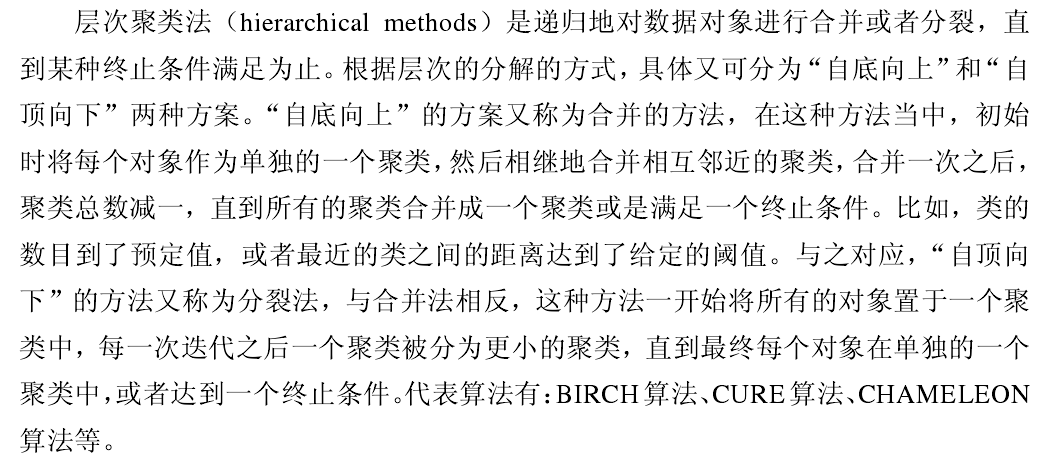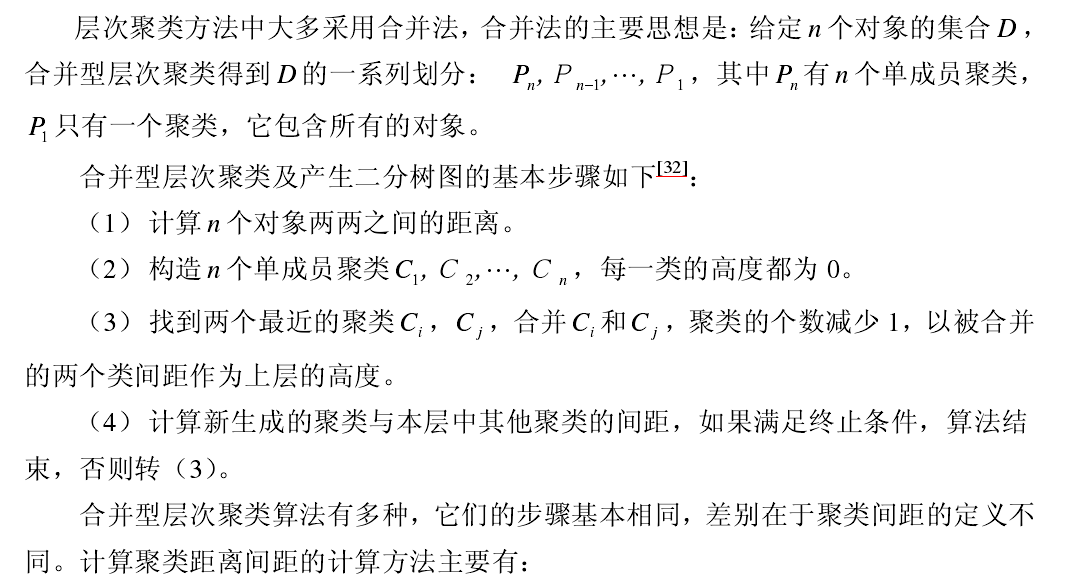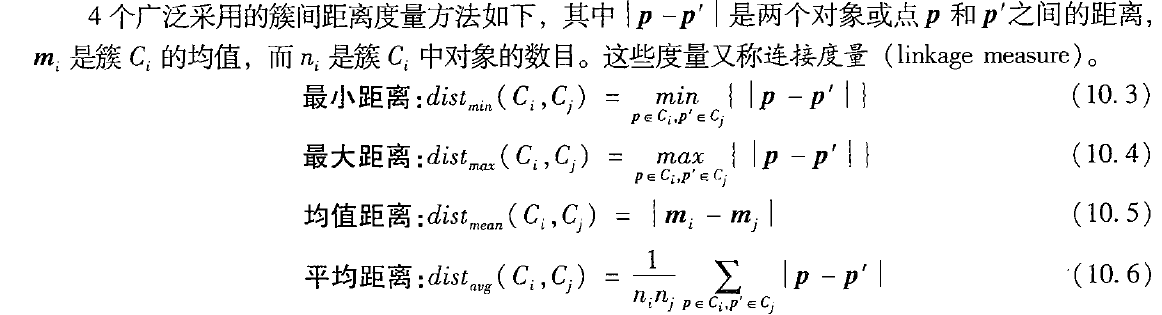AGNES算法（自底向上层次聚类）

AGNES(Agglomerative Nesting) 是凝聚的层次聚类算法，如果簇C1中的一个对象和簇C2中的一个对象之间的距离是所有属于不同簇的对象间欧式距离中最小的，C1和C2可能被合并。这是一种单连接方法，其每个簇可以被簇中的所有对象代表，两个簇之间的相似度由这两个簇中距离最近的数据点对的相似度来确定。

算法描述：

输入：包含n个对象的数据库，终止条件簇的数目k

输出：k个簇

（1）       将每个对象当成一个初始簇

（2）       Repeat

（3）                根据两个簇中最近的数据点找到最近的两个簇

（4）                合并两个簇，生成新的簇的集合

（5）       Until达到定义的簇的数目

算法性能：

（1）       简单，但遇到合并点选择困难的情况。

（2）       一旦一组对象被合并，不能撤销

（3）       算法的复杂度为O(n的平方)，不适合大数据集
DIANA（Divisive Analysis）算法属于分裂的层次聚类，首先将所有的对象初始化到一个簇中，然后根据一些原则（比如最邻近的最大欧式距离），将该簇分类。直到到达用户指定的簇数目或者两个簇之间的距离超过了某个阈值。

DIANA用到如下两个定义：

（1）       簇的直径：在一个簇中的任意两个数据点都有一个欧氏距离，这些距离中的最大值是簇的直径

（2）       平均相异度（平均距离）：

算法描述：

输入：包含n个对象的数据库，终止条件簇的数目k

输出：k个簇，达到终止条件规定簇数目

（1）       将所有对象整个当成一个初始簇

（2）       For ( i=1;i!=k;i++) Do Begin

（3）         在所有簇中挑选出具有最大直径的簇；

（4）           找出所挑出簇里与其他点平均相异度最大的一个点放入splinter group，剩余的放入old party中。

（5）           Repeat

（6）             在old party里找出到splinter group中点的最近距离不大于old party中点的最近距离的点，并将该点加入splinter group

（7）           Until 没有新的old party的点被分配给splinter group；

（8）       Splinter group 和old party为被选中的簇分裂成的两个簇，与其他簇一起组成新的簇集合

（9）       END

算法性能：

缺点是已做的分裂操作不能撤销，类之间不能交换对象。如果在某步没有选择好分裂点，可能会导致低质量的聚类结果。大数据集不太适用。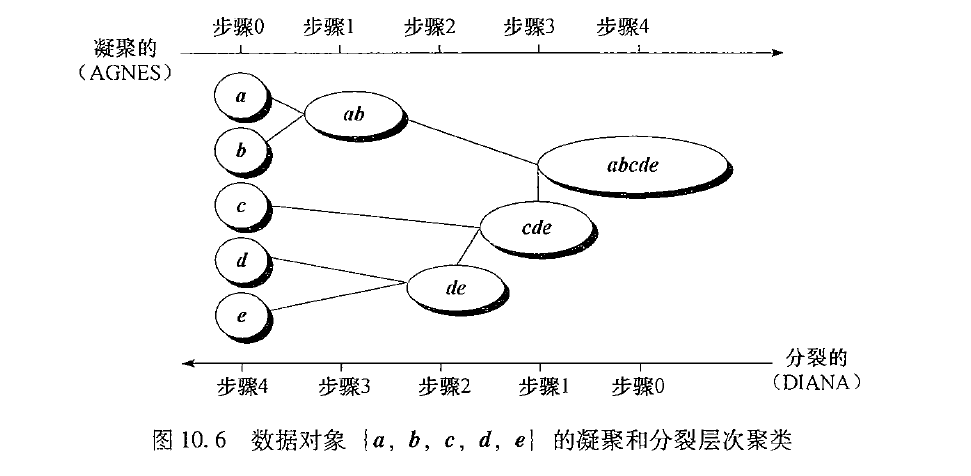层次聚类算法的分析：

层次聚类法的优点是可以通过设置不同的相关参数值，得到不同粒度上的多层次聚类结构；在聚类形状方面，层次聚类适用于任意形状的聚类，并且对样本的输入顺序是不敏感的。 层次聚类的不足之处是算法的时间复杂度大，层次聚类的结果依赖聚类的合并点和分裂点的选择。并且层次聚类过程最明显的特点就是不可逆性，由于对象在合并或分裂之后，下一次聚类会在前一次聚类基础之上继续进行合并或分裂，也就是说，一旦聚类结果形成，想要再重新合并来优化聚类的性能是不可能的了。聚类终止的条件的不精确性是层次聚类的另一个缺点，层次聚类要求指定一个合并或分解的终止条件，比如指定聚类的个数或是两个距离最近的聚类之间最小距离阈值。

展开全文DIANA 自底向上 自顶向下
• 首先将每个对象作为一个簇，然后合并这些原子簇为越来越大簇，直到所有对象都在一个簇中，或者某个终结条件被满足，绝大多数层次聚类方法属于这一类，它们只是在簇间相似度定义上有所不同。 2分裂层次聚类...
• 首先将每个对象作为一个簇，然后合并这些原子簇为越来越大簇，直到所有对象都在一个簇中，或者某个终结条件被满足，绝大多数层次聚类方法属于这一类，它们只是在簇间相似度定义上有所不同。 2分裂层次聚类...
• ## 层次聚类算法

千次阅读 2015-10-19 17:17:07
一、算法介绍 将物理或抽象对象集合分成由类似对象组成多个类过程被称为聚类。由聚类所生成簇是一组数据对象集合，类中对象...不同于K-means这种划分式聚类算法，层次聚类最终生成聚类层次，也就是数据挖掘 算法 机器学习
• 层次聚类中，起初每一个实例或观测值属于一类，聚类就是每一次把两...直到包含所有观测值的类合并成单个的类为止在层次聚类中，主要的区别是它们对类的定义不同(步骤(b))，五种常见的聚类方法的定义和其中两类之间的...
• 1.概要： 层次聚类方法将数据对象...绝大多数层次聚类方法属于这一类 分裂层次聚类：采用自顶向下策略，首先将所有对象置于一个簇中，然后逐渐细分为越来越小簇，直到每一个对象自成一个簇，或者达到某个终止条件，算法 python 数据挖掘
• 聚类大致分为以下两类： 模糊聚类：对象与类的从属关系是确定的；属于或者不属于；...凝聚式层次法：指的是先将每个观测归于一类，然后每次将最相似的两个大类合并成一个新的类，直至所有的观测成为一...
• 层次聚类算法的定义为：通过某种可计算方法的相似度测度计算节点（分析对象）之间的相似性，并按相似度由高到低排序，逐步重新连接个节点，得到一个单聚类结果。 简单描述就相当于：三角形的重点、中点、及内心这种...python
• 聚类方法一般可以分为层次聚类与非层次聚类两种。其中层次聚类算法又可以分为合并法与分解法；同样非层次聚类算法也可以分为多种，常用有K-means算法。这篇博客先来实现层次聚类算法中合并法，我会在下一篇博文...机器学习 java
• 层次聚类(Hierarchical Clustering)是一种聚类算法，属于无监督学习。层次聚类通过计算不同类别数据点间相似度来创建一棵有层次嵌套聚类树。在聚类树中，不同类别原始数据点是树叶节点，树顶层是一个聚类...机器学习
• github链接：[gdutthu/Statistical-learning-method]本人csdn博客链接：https://blog.csdn.net/zhanzi1538/article/details/106881259算法...3、k-means属于聚类方法，得到聚类结果是平坦，非层次（通俗...
• 统计学习方法-聚类方法-读书笔记1、前言2、聚类基本概念2.1相似度或距离2.2类或簇2.3类与类之间距离3、层次聚类4、K均值聚类 1、前言 聚类事针对给定样本，依据他们特征和相似度或距离，将其归并到若干个类...机器学习
• 聚类算法就属于无监督算法一种。聚类算法可以分为两大类： 分割型聚类（每个样本点只能在一个类别中） k-means/k-medoids聚类 层次聚类 树状数据结构，每一层是一种分类方法。 二：聚类算法应用 ...
• 我们正在执行K-Means聚类和层次聚类机制，最后，我们实现了一个混淆矩阵，以实现并确定这两种算法中最高准确性。 在这里，我们考虑使用机器学习机制根据自变量来预测客户是否可以购买产品类别。 这项工作为您...
• 聚类算法从原理上大致课划分为3类：（1）基于原型的聚类（2）基于密度的聚类（3）基于层次的聚类，而在每一类下面又有好多算法，今天我要介绍就是k均值聚类算法，它属于”基于原型聚类“这一类别。（ 先提个问题：...
• 在机器学习中聚类属于无监督学习，常用算法很多，书中介绍了两种：层次聚类（hierarchical clustering）和 k均值聚类（k-means clustering）. 相似度和距离： 如何定义样本之间相似度或关系，这就需要一些度量...机器学习
• ## 聚类算法总结

千次阅读 2018-06-08 23:14:11
聚类算法概念： 聚类就是按照某个特定标准(如距离准则)把一个数据集聚成不同...聚类算法研究也是一个很大家族，比较常见有基于划分的聚类方法、基于层次的聚类方法、基于密度的聚类方法、基于网络的聚类方法...
• 支持向量机-《统计学习方法》学习笔记1 概述2聚类基本概念2.1 相似度或距离2.2 类或簇2.3 类... 常用聚类算法有层次聚类和k均值聚类。层次聚类又分为聚合和分裂两种方法。本文章主要介绍这两种聚类算法。 2聚类矩阵 算法 机器学习 数据挖掘
• 聚类大致分为以下两类： 模糊聚类：对象与类的从属关系是确定的；属于或者不属于；...凝聚式层次法：指的是先将每个观测归于一类，然后每次将最相似的两个大类合并成一个新的类，直至所有的观测成为一...
• Canopy算法是2000年由Andrew McCallum, Kamal Nigam and Lyle Ungar提出来，它是对k-means聚类算法和层次聚类算法预处理。众所周知，kmeans一个不足之处在于k值需要通过人为进行调整，后期可以通过肘部法则...
• 题目: 1 用SPSS软件对一个班同学数学水平进行聚类分析。聚类依据是第一次数学考试成绩和...8.2 层次聚类分析中Q型聚类 8.2.1 统计学上定义和计算公式 1. 连续变量样本距离测试方法 2. 连续变量
• 文章目录聚类性能度量距离计算原形聚类K-means 聚类高斯混合聚类密度聚类层次聚类降维贝叶斯分类器 本篇文章主要是参考周志华老师《机器学习》（西瓜书）其中部分章节，做一个知识快速整理和简单理解，因为本...
• 平坦聚类/分割聚类【直接将样本分割为多个不相交子集】、层次聚类【通过构造具有层级树形结构，在不同层次上对样本进行分割】 K-means聚类 起源于信号处理，是一种应用较广泛聚类分析方法，目标是将n个样本...python 机器学习
• 传统的聚类分析计算方法主要有如下几种：划分方法层次方法、基于密度的方法、基于网格的方法、基于模型的方法等。其中K-Means算法是划分方法中的一个经典的算法。 1、聚类 “类”指的是具有相似性的集合，聚类是...深度学习
• 重点掌握 层次聚类，并且能够总结出几种经典层次聚类方法来讲。 10.1聚类分析（原书P288） 概念：把数据对象划分成子集过程 是统计学分支，主要集中在基于距离聚类分析 。 分类属于监督学习。 聚类...数据挖掘
• 这两天先对于简单聚类算法有一个初步了解分类大致可以分为7类层次化聚类算法划分式聚类算法基于模型聚类算法基于密度聚类算法基于网络聚类算法基于约束聚类算法基于模糊的聚类方法首先来看一下聚类...